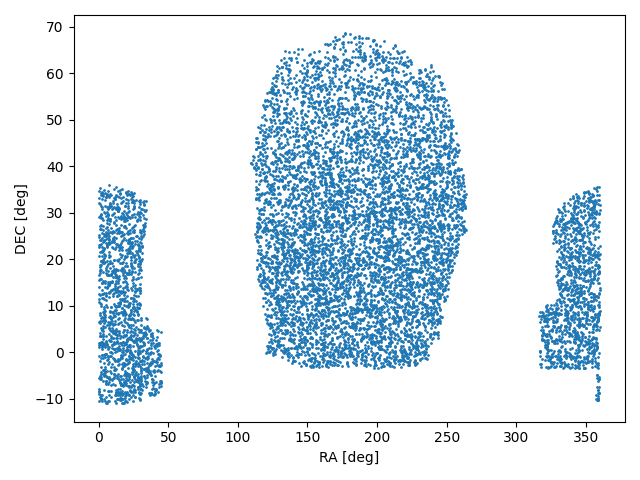# RandomSDSS’s documentation!Generate random points in Sloan Digital Sky Survey (SDSS) DR8 to DR16 footprints.

This is a small wrapper around the package pymangle that facilitates the creation of random points in the SDSS fields. I included SDSS polygons for its different data releases (DR8 to DR16).

## Basic Usage

```import matplotlib.pyplot as plt
import randomsdss

dr12 = randomsdss.DR12(catalog="BOSS")
ra, dec = dr12.sky_random(size=10_000)

plt.figure()
plt.scatter(ra, dec, s=1)
plt.xlabel('RA [deg]')
plt.ylabel('DEC [deg]')
```Alternatively, you can get the same result without the need to instantiate an object using:

```ra, dec = randomsdss.sky_random(dr="DR12", catalog="BOSS", size=10_000)
```

If you also need a random redshift distribution you can provide a sample of redshifts and a random set will be generated from the underlying Probability Density Function (PDF):

```z = randomsdss.z_random(z_array, size=10_000)
```

The z_random is a complementary function since it does not use any information regarding the SDSS catalogs, only the provided redshift array.

Author
Martin Chalela (E-mail: tinchochalela@gmail.com)

## Repository and Issues

https://github.com/mchalela/RandomSDSS

Contents: